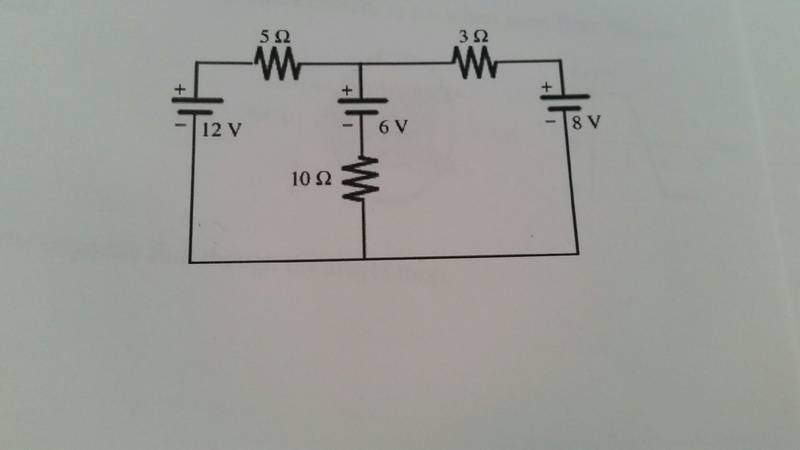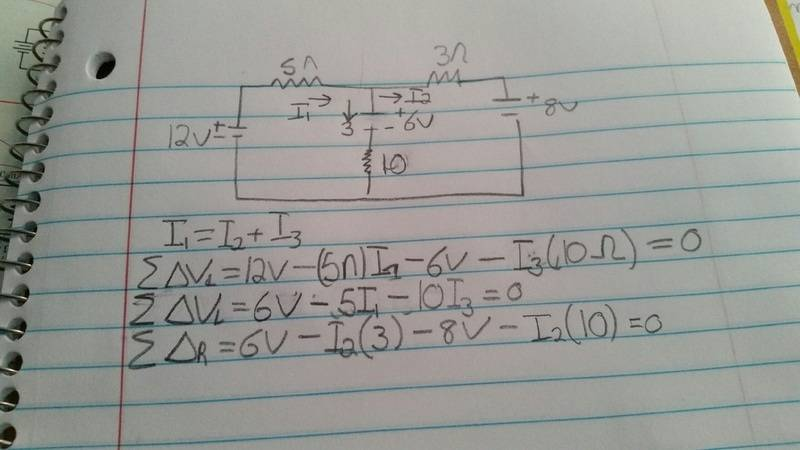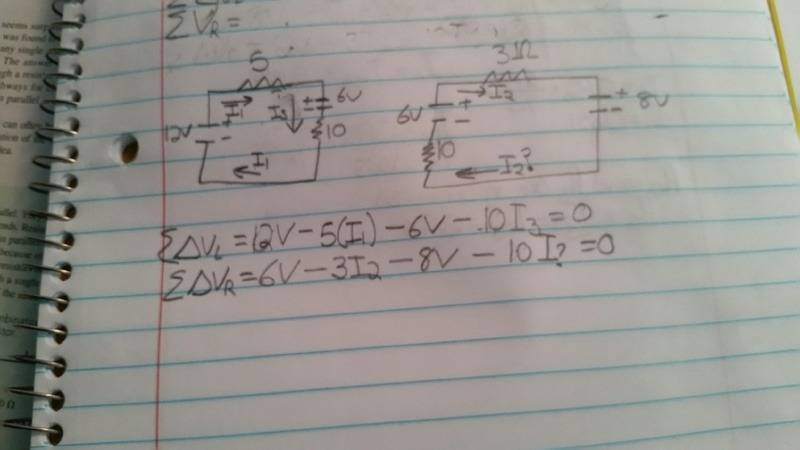# 3 battery resistor circuit

## Homework Statement

Find the current through and power dissipated by each resistorV=I/R
ΣIin=∑Iout

## The Attempt at a SolutionDid I set up the loop laws correctly and where do I go from here?

gneill
Mentor
Your first loop equation looks fine. I can't picture what your second loop is from the equation that you wrote, but I can say that it is incorrect. Your third loop equation has an error in the last term: current I2 does not pass through the 10 Ω resistor.

Can you label the nodes in your diagram and state your loop paths?

Hint: You only need enough loops so that each component is included in at least one of the loops. In this problem you can get away with two loop equations.

•ReidMerrill
Since the 10Ω resistor is already in the first loop do I even need to include it in the second?

gneill
Mentor
Since the 10Ω resistor is already in the first loop do I even need to include it in the second?
Every loop must complete a circuit (be a closed path) and some components will be shared by more than one loop; that's expected. You must include a term for every component in the "KVL walk" that you take around given loop in order for KVL to hold.

•ReidMerrillI'm not sure if the current going through the 10Ω resistor is different/needs a different name
I'm also going to see if starting from the 8V battery makes more sense to me.

gneill
Mentor
Once you've labeled a current though a component, that's the current that is associated with it. It doesn't change if you apply KVL to different loops that happen to share that component. So ##I_3## flows through the 10 Ω resistor, in the same direction as you chose it, for each KVL equation that contains that resistor. So just be careful of the signs of the terms: if you "KVL walk" over a resistor against the flow of the current, then you will "see" a potential rise instead of a drop.

In your second equation above, you are "walking" clockwise around the loop, so when you traverse the 10 Ω resistor you are proceeding upwards, against the flow of ##I_3##. Will you see a potential drop or a potential rise as a result?

•ReidMerrill